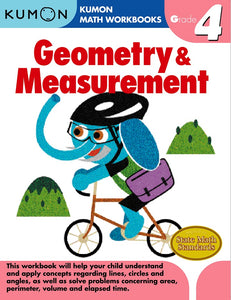Kumon

# Kumon Grade 4: Geometry & Measurement

Regular price \$13.80 \$0.00 Unit price per
Shipping calculated at checkout.

Your child will progress gradually through our activities and master area, volume, circumference, and diameter. Children learn to measure angles with a protractor and calculate decimal and fraction problems with ease.

Read and write fractions on a number line
Read and write decimals on a number line
Understand and apply concepts of lines, angles, and circles
Draw two-dimensional figures
Measure and find the area and perimeter of rectangular shapes using appropriate units.
Measure volume using standard cubic units.
Measure surface area of cubes and rectangular prisms by adding up the area of the faces
Calculate elapsed time in hours and half hours
Calculate elapsed time in days and weeks